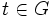# Approximate normalizer

Jump to: navigation, search
This is a variation of normalizer|Find other variations of normalizer |

## Contents

This article defines a term that has been used or referenced in a journal article or standard publication, but may not be generally accepted by the mathematical community as a standard term.[SHOW MORE]

## Definition

### Definition with symbols

Let$G$ be a group and$g \in G$ be an element. Then, the approximate normalizer of$g$, denoted as$N_G^*(g)$ is defined as the set of all elements$t \in G$ for which there exist nonzero integers$m,n$ such that$t^{-1}g^mt = g^n$.

The approximate normalizer of any element is a subgroup of the whole group. It equals the whole group if the element has finite order (viz, is a torsion element). Thus, the notion makes sense to study only for elements of infinite order.